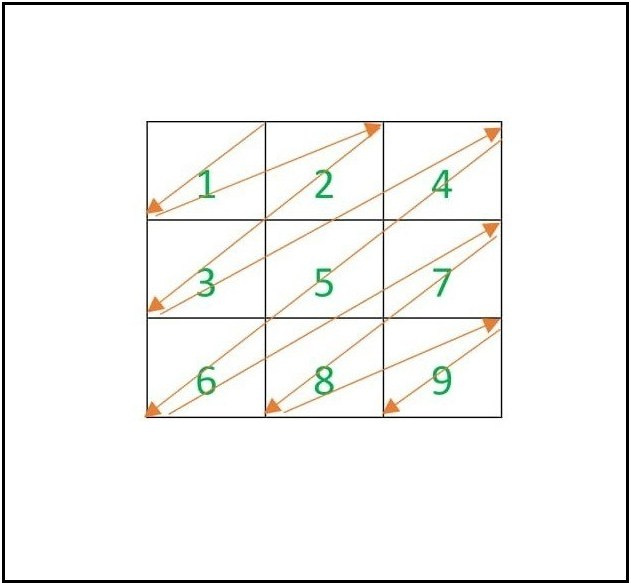# Arrange numbers 1 to N^2 in a Zig-Zag Matrix in ascending order

Given a positive integer N, the task is to print an N × N zig-zag matrix consisting of numbers from 1 to N2, such that the ZigZag traversal of the matrix yields the number in ascending order.

Examples:

Input: N = 3
Output:
1 2 4
3 5 7
6 8 9
Explanation:Input: N = 4
Output:
1 2 4 7
3 5 8 11
6 9 12 14
10 13 15 16

Approach:
The required matrix can be broken down into two right angle triangles.

• An upside down right angled triangle(considered as upper triangle).
• A normal right angled triangle(considered as lower triangle).

The idea is to iterate two nested loop to fill the upper triangle with respective values. Then iterate two nested loop again to fill the lower triangle with respective values. After the above two operations print the desired matrix.

Below is the implementation of the above approach:

## C++

 `// C++ program for the above approach ` `#include ` `using` `namespace` `std; ` ` `  `// Function to print the pattern ` `void` `printPattern(``int` `n) ` `{ ` `    ``// N * N matrix to store the ` `    ``// values ` `    ``int` `arr[n][n]; ` ` `  `    ``arr = 1; ` ` `  `    ``// Fill the values of ` `    ``// upper triangle ` `    ``for` `(``int` `i = 0; i < n; i++) { ` ` `  `        ``if` `(i > 0) { ` `            ``arr[i] = arr[i - 1] + i + 1; ` `        ``} ` `        ``for` `(``int` `j = 1; ` `             ``j < n - i; j++) { ` ` `  `            ``arr[i][j] = arr[i][j - 1] + i + j; ` `        ``} ` `    ``} ` ` `  `    ``// Fill the values of ` `    ``// lower triangle ` `    ``arr[n - 1] = arr[n - 1] + 1; ` `    ``int` `div` `= 0; ` ` `  `    ``for` `(``int` `i = 2; i < n; i++) { ` ` `  `        ``div` `= n - 2; ` `        ``for` `(``int` `j = n - i; ` `             ``j < n; j++) { ` ` `  `            ``if` `(j == n - i) { ` `                ``arr[i][j] = arr[i - 1][j + 1] ` `                            ``+ 1; ` `            ``} ` `            ``else` `{ ` `                ``arr[i][j] = arr[i][j - 1] ` `                            ``+ ``div``; ` `                ``div``--; ` `            ``} ` `        ``} ` `    ``} ` ` `  `    ``// Print the array ` `    ``for` `(``int` `i = 0; i < n; i++) { ` ` `  `        ``for` `(``int` `j = 0; j < n; j++) { ` ` `  `            ``cout << arr[i][j] << ``" "``; ` `        ``} ` `        ``cout << ``"\n"``; ` `    ``} ` `} ` ` `  `// Driver Code ` `int` `main() ` `{ ` `    ``// Given size of matrix ` `    ``int` `N = 4; ` ` `  `    ``// Function Call ` `    ``printPattern(N); ` `    ``return` `0; ` `} `

Output:

```1 2 4 7
3 5 8 11
6 9 12 14
10 13 15 16
```

Time Complexity: O(N2)
Auxillary Space: O(N2)

Attention reader! Don’t stop learning now. Get hold of all the important DSA concepts with the DSA Self Paced Course at a student-friendly price and become industry ready.

My Personal Notes arrow_drop_upCheck out this Author's contributed articles.

If you like GeeksforGeeks and would like to contribute, you can also write an article using contribute.geeksforgeeks.org or mail your article to contribute@geeksforgeeks.org. See your article appearing on the GeeksforGeeks main page and help other Geeks.

Please Improve this article if you find anything incorrect by clicking on the "Improve Article" button below.